# Perfection Ain't So Perfect

Brian Alspach

Poker Digest Vol. 1, No. 9, November 23 - December 3, 1998

Recently there were a few messages on the newsgroup RGP about perfect shuffles. There was a statement claiming that after eight perfect shuffles the deck returns to its original order. Let's take a look at this claim. We shall see how the right mathematical notation can help considerably towards determining an answer to a problem.

What is a perfect shuffle? The first step consists of cutting the deck so that each pile has precisely 26 cards. This is not an easy task for a human to perform consistently. The second step consists of shuffling the cards so that the cards of the two halves of the deck interleave alternately. That is, the top card of the right half becomes the top card, then the top card of the left half becomes the second card, the second card of the right half becomes the third card, the second card of the left half becomes the fourth card, and so on. This also is not a simple physical task to perform. Most people have clumps as the two halves alternate. Perci Diaconis reportedly is able to perform successive perfect shuffles. He also has done interesting theoretical work on card shuffling.

The proper mathematical tool for examining shuffling is the notion of a permutation. A permutation of a set of objects can be thought of as a rearrangement of the objects. For example, suppose we have four objects 1,2,3,4 and we rearrange them as 2,1,3,4. A good way to think of this is to use the language of functions. Letting f be the function, we have f(1)=2, f(2)=1, f(3)=3, and f(4)=4 in the preceding example. Given a function f on a finite set S, there are two characteristics which make the function a permutation. First, f cannot send two different objects to the same object, that is, if f(i) = f(j), then i = j must hold. Second, for each object k in S, there is some i in S for which f(i) = k.

Suppose that f is a permutation of the set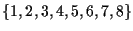. We want to devise a notation that tells us how f permutes the objects. One notation, called the range notation, displays the elements in the order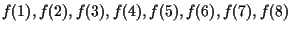. Thus, you can see immediately how the permutation is moving objects. So 3,7,2,5,8,1,4,6 means the permutation moves 1 to 3, 2 to 7, 3 to 2, and so on.

What we want to know is how many perfect shuffles must be done in succession before the deck is back to its original order. Let's interpret this in terms of a permutation of the positions of the cards. The permutation leaving everything as is, that is, nothing is rearranged is called the identity permutation. The identity permutation is denoted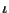. If we perform the permutation f twice in succession, we use the notation f2 to denote the resulting permutation. Likewise, f3 denotes the permutation f performed three times in succession. We are interested in the smallest k such that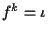. This value of k is called the order of f.

If one takes an arbitrary permutation f and displays it in range notation, it is not easy to see what the order of f is. One simply has to keep iterating the permutation until the identity is reached. That can involve a lot of computation and is amenable to making errors. Let's introduce another notation for permutations which allows the order to be computed almost instantly, and then apply it to the permutation describing a perfect shuffle. This notation is called cyclic notation. What one does in cyclic notation is to write down one of the elements being permuted; call it z. Then one writes down f(z) the element to which f moves z. Now one writes down f(f(z)) the element to which f moves f(z). Then one writes down f(f(f(z))) the element to which f moves f(f(z)). One continues in this way until reaching the element w which f sends back to the starting element z. Then enclose this string of elements with parentheses. If there are elements not included within the parentheses, start over with a new element. Continue until all elements have been included.

Let's consider two examples to illustrate the preceding definition. Return to the first permutation above whose range notation is 2,1,3,4. In cyclic notation we obtain (1 2)(3)(4) because the permutation sends 1 to 2 and then 2 back to 1. It sends 3 to itself and 4 to itself. You can see easily what a permutation is doing in cyclic notation because you read the action from left to right in each parentheses until reaching the last entry whichs is permuted back to the element beginning the expression within the parentheses. As a second example, consider the permutation 3,7,2,5,8,1,4,6 above. In cyclic notation we obtain (1 3 2 7 4 5 8 6). Check that you believe they are the same permutation. Each of the expressions contained in parentheses is called a cycle of the permutation. If the cycle has length 8, as above, it is easy to see eight iterations of the permutation give you the identity. This means that if you iterate it any multiple of eight times you obtain the identity. Hence, if the permutation is (1 3 5)(2 4 7 6), you must iterate a multiple of 3 and a multiple of 4 to get the identity. In this case, 12 iterations is the first time you obtain the identity. Therefore, it is not hard to see that once you have the cycle lengths for the permutation, you simply take the least common multiple of the cycle lengths to compute the order.

The permutation of positions for a perfect shuffle is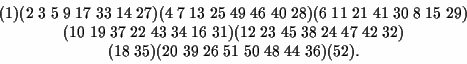Since all cycle lengths are 8 or have 8 as a multiple, the order of this permutation is 8 and the claim at the beginning of the article is correct.

A variation on this is to have the cards of the left half start first so they end up in the odd positions. The permutation is very different now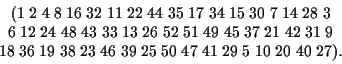This means you will not return to the original order until you have performed 52 of these shuffles. So a small variation on the shuffle makes a huge change in the order.

Even though the first shuffle described above is called a perfect shuffle, the outcome for the order of the cards is completely predictable which might be viewed as far from perfect with regard to randomizing the cards. There is a lot which can be said about shuffles.

Home | Publications | Preprints | MITACS | Poker Digest | Poker Computations | Feedback
website by the Centre for Systems Science
last updated 20 August 2001